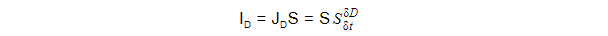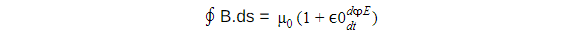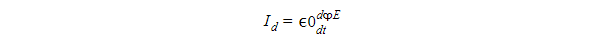• Call Now

1800-102-2727•

# Displacement current

The rate of change of the electric displacement field is defined as displacement current. When described using Maxwell's Equation, it is easy to grasp.

## Maxwell-Ampere Law

Electricity and magnetism are important aspects of physical science. Because electricity and magnetism are inextricably connected, they are classified as a single topic known as electromagnetism. When working with a current-carrying wire, we may see a glimpse into electromagnetic. When an electric current flows through a wire, it creates a magnetic field that surrounds the wire or any conductor it passes through.
Conduction current is a type of current that flows through a conductor and is created by the passage of electrons through the conductor. This sort of current is commonly seen in our daily lives. There is also displacement current, which is a kind of current. Displacement current differs from conduction current in that it does not entail the movement of electrons. The displacement current is crucial to the propagation of electromagnetic waves.
Before Maxwell created an equation, another scientist, Andre-Marie Ampere, created a renowned equation known as Ampere's law. When a conduction current (I) flows through a closed-loop, a magnetic field (B) is created around the closed-loop, according to Ampere's law.

The Ampere Maxwell equation is as follows:

∮B.ds = μₒI

Where u 0 denotes the permeability of open space.

Ampere's law applies in every scenario where there is a constant source of conduction current. When Ampere's law is employed to calculate the figures in written form, the issue emerges. Let's look at an electric circuit using a capacitor to see how this works. A voltage source is linked to charge a capacitor, as shown in the picture below. Positive charges (Q+) and negative charges (Q-) build on the capacitor's opposing plates.
Displacement current expression
The displacement current equation is given by,Where,

• S denotes the area of the capacitor's plate.
• The displacement current is denoted by ID.
• The density of displacement current is denoted by JD.
• D Is it connected to the electric field? D=εE
• ε is the permittivity of the medium located between the plates.

Electric current passes across the circuit wires during the charging and discharging of the capacitor. However, no current flows between the capacitor's plates. According to Ampere's law, there should be no magnetic field between the plates because there is no current, yet there is one. To address this restriction of Ampere's law, Maxwell added a term to the equation of Ampere's law.

Conduction current, whether constant or variable, always generates a magnetic field around the conductor through which it flows. Maxwell predicted in the 91st century that the magnetic field will continue to exist even in the absence of conduction current and that the magnetic field may be connected with the changing electric field. Maxwell's hypothesis was empirically proven in the years that followed.

Because the magnetic field is related to the electric field, the general displacement current formula is as follows:This is the generalised formula for the Maxwell-Ampere law.

## Current Definition of Displacement

Maxwell's contribution to Ampere's law is the displacement current (ID). Let's look at the displacement current formula first to have a better understanding of the topic.The following equation is made up of two multiplying terms: the permittivity of empty space, and the derivative of electric flux with respect to time. The time rate change of the passage of an electric field through a surface is defined as electric flux. When we take the derivative of electric flux, we obtain the rate of change of the electric field over time in a particular region.Talk to our expert
Resend OTP Timer =
By submitting up, I agree to receive all the Whatsapp communication on my registered number and Aakash terms and conditions and privacy policy